# Analysis ToolPak In ExcelArticle byJeevan A Y## What Is Analysis ToolPak In Excel?

Analysis ToolPak in Excel is an Excel Add-in that comes with a variety of data analysis features and techniques to save a lot of time for users. It offers data analysis for financial, statistical, and engineering data and assists decision-making.

Analysis toolpak contains various kinds of calculations in its armories like Descriptive Statistics, ANOVA, Rank and Percentile, Correlation, Regression, etc.

###### Key Takeaways
• Analysis ToolPak offers a variety of statistical options, and we have learned about applying the ANOVA test, Correlation, Rank & Percentile, and Descriptive Statistics.
• The ANOVA test will let us know whether our null hypothesis is true or not. The higher the value of p, the more likely the data mean is the same.
• Correlation allows us to find the relationship between two or more variables. Correlation values range from -1 to 1. Less than 0 value indicates the negative Correlation, and greater than 0 value indicates the positive Correlation.
• Rank & Percentile will sort the data in descending order automatically while returning the output range.
• Descriptive Statistics allows the user to get through many statistical calculations in one go.

### How To Add Analysis ToolPak In Excel?

Analysis ToolPak in Excel will not be found in an Excel workbook by default. We must add or enable it, then we can view it under the Data tab, as shown in the following image.

The steps to enable Analysis Toolpak are listed as follows.

1. Select the File tab.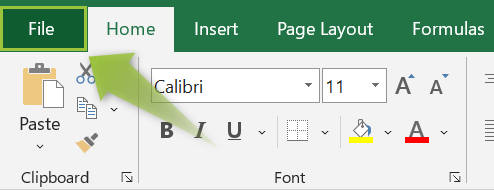2. Click the “Options” tab.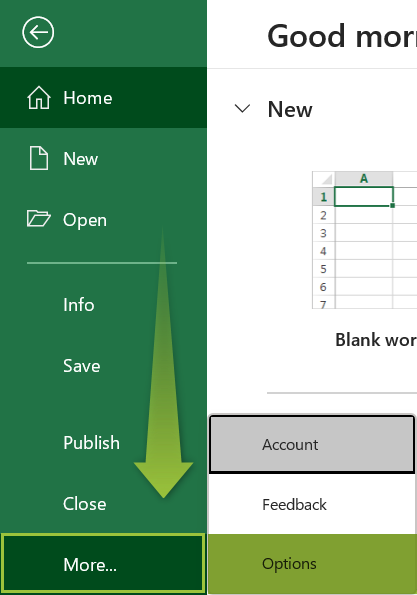3. This will open the “Excel Options” window. Click the “Add-ins” option on the left.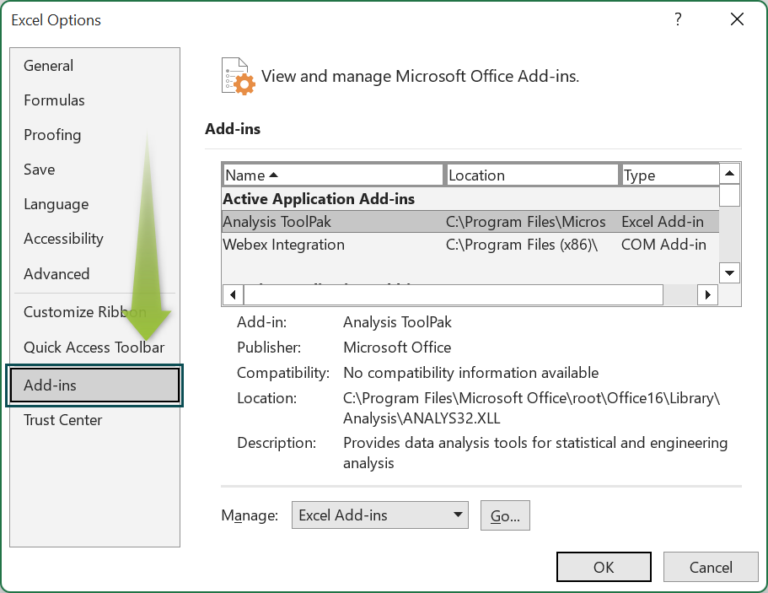4. In the “Manage” drop-down, choose the “Excel Add-ins” option, and click “Go…”.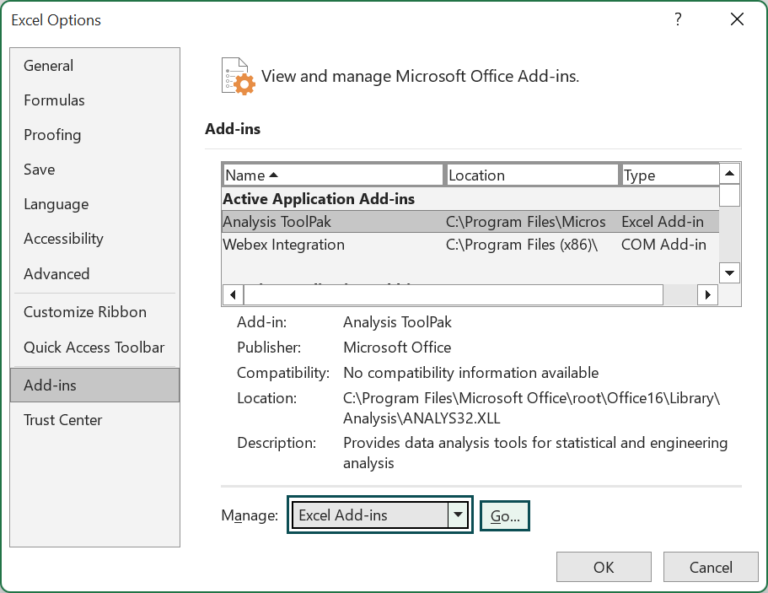5. This will open the “Add-ins” window. Check the “Analysis ToolPak” checkbox, and click “OK”.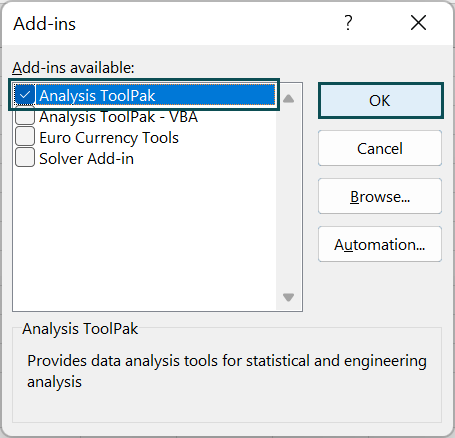Now, we will see the “Data Analysis” option under the Data tab.### How To Use Analysis ToolPak In Excel?

We can use the Analysis ToolPak in Excel using the following functions,

• Anova.
• Correlation.
• Rank and Percentile.
• Descriptive Statistics.

#### #1 – ANOVA Single Factor

ANOVA stands for “Analysis of Variance”. ANOVA is used to test different data, and identify the difference between their average. Sample data is used to infer the properties of the larger data set or an entire population.

For instance, students from different colleges in the city take the same exam to check if any one of the colleges is making a significant difference in the result of the examination.

We have the following top 10 student marks data from 4 colleges to compare the scores of these colleges.

In this scenario, colleges are our independent variable, and marks are the dependent variable.

The null hypothesis is that there is a statistical significance between the scores of the 4 colleges. Let us test this null hypothesis by testing the P-value using single-factor ANOVA.

The steps to test whether the mean scores of students from the 4 different colleges are different using the Anova: Single Factor are as follows:

• Step 1: Select the Data tab → go to the “Analysis” group → click the “Data Analysis” option.
• Step 2: The “Data Analysis” window opens. Choose the first option, “Anova: Single Factor”, and click “OK”.
• Step 3: The “ANOVA: Single Factor” window opens, as shown below.
• Step 4: For the Input Range, choose the values from E1:E11.
• Step 5: In the group by option choose “Columns” because our data is structured vertically.
• Step 6: Since we are using the column headers in the Input Range, check the box “Labels in the first row”, that helps to interpret the data easily.
• Step 7: Excel, by default, uses the Alpha value as 0.05, which is good. Alpha is the significance level, which we can change accordingly to our data significance.
• Step 8: Under output options, choose “Output Range:” and choose the cell address where we want to arrive at the result of the ANOVA test.
• Step 9: Click on “OK”, and we will get the following ANOVA result, as shown below.
• Interpretation of the Result of the ANOVA Single Factor:

From the above summary table of the ANOVA test, we can see that means (average) ranges from 68.5 for the first college to 84.7 for college 4.

There is a significant gap between the mean scores of the colleges. The p-factor value of 0.3448 is greater than the significance level value of 0.05, so we can accept the null hypothesis of there being a significant difference between the means of the scores of each college.

#### #2 – Correlation

Correlation is the statistical method used to find the relationship between two variables. For instance, let us take an example of height and weight Correlation.

Correlation calculation gives us the values between -1 and 1. We can use this Correlation number to interpret the result as follows:

• If the Correlation value is less than zero, then it means that, if the height increases, the weight decreases, or if the weight increases, then the height decreases.
• If the Correlation value is greater than 0, then it means, if the height increases, then weight also increases, and if the weight decreases, then height also decreases. This is called a positive Correlation.
• When the Correlation value is closer to 1, the positive Correlation is strong. Hence, the Correlation value of 0.8 predicts a strong Correlation between height and weight.

Note: This example is limited to the Pearson Correlation Coefficient.

For example, we have the following sales data for air coolers w.r.t temperature in the months and advertisement costs.

The steps to find out the Correlation between temperature, air coolers sold, and the advertisement cost are as follows:

• Step 1: Select the Data tab → go to the “Analysis” group → click the “Data Analysis” option.
• Step 2: The “Data Analysis” window opens. Choose the first option, “Correlation”, and click “OK”.
• Step 3: In the Correlation window, choose the Input Range: as B1:D13.
• Step 4: Choose Columns under “Grouped By:”, and click “OK”.
• Step 5: Next, check the box “Labels in First Row”. This will help us in read the summary result in the same headers as the data table.
• Step 6: Select the Output Range: as any of the empty cells, here, cell F1 is selected.
• Step 7: Click on “OK”, and we will get the Correlation results.
• Interpretation of the Result of Correlation:

The result of the Correlation is the intersection of the rows and columns.  Wherever the intersection of the row and column is the same, we have the Correlation value as 1.

For example, Temp vs Temp, we have 1.

Next, the Correlation value between ACs sold and Temp is 0.72 (rounded to 2 decimal places).

This means that when the temperature increases, the number of ACs sold increases too, and this Correlation is a positive Correlation and quite significant.

Next, the Correlation value between Temp and Adv Cost is 0.53 (rounded to 2 decimal places).

Advertisement cost is a positive Correlation, however, not as significant as the previous Correlation of Temp & AC’s Sold. In this way, we can find the Correlation between two or more factors.

#### #3 – Rank and Percentile

Rank and Percentile are the two statistics done together as one to rank and find the percentile position of each data point.

For instance, we have the following student’s score in Excel.

The steps to find the Rank and Percentile for the above exam scores are as follows:

• Step 1: Select the Data tab → go to the “Analysis” group → click the “Data Analysis” option.
• Step 2: The “Data Analysis” window opens. Choose the first option, “Rank and Percentile”, and click “OK”.
• Step 3: In the “Rank and Percentile” window, choose the Input Range: from B1:B11.
• Step 4: Choose Columns under “Grouped By:”, and check the box “Labels in first row”.
• Step 5: Choose the Output Range: as any empty cells, here, cell D1 is selected, and click “OK”.

We will get the result as shown in the following image.

• Interpretation of the Result of Rank and Percentile:

We have 4 columns as an output result when we have applied Rank and Percentile data analysis from Analysis Toolpak.

The first column is “Point”, i.e., the position of the score in the original data table. For instance, score 67 got the point as 1, so score 67 is the first score in the data table.

The rank column applies the ranking for each score. Ranking ranges from 1 to 10, with the highest ranked as 1 and the lowest ranked as 10. For example, score 97 is the rank 1, and score 45 ranked is 10.

Next, we have the percent column that says at each data point what pentile scores are less than or equal to the current score. For instance, the score 66’s percent is 55.5%, i.e., 55.5% of the scores are less than or equal to the score of 66.

#### #4 – Descriptive Statistics

In statistics, we have many calculations like AVERAGE, MIN, MAX, RANGE, STDDEV, etc. From the given data set, it is difficult to do each calculation one after the other. However, Descriptive Statistics allows us to perform the following calculations.

1. Measures of Frequency
• Min, Max, Count, Percent, and Frequency. It is used to show how often something occurs.

2. Measures of Central Tendency

• Mean, Mode, and Median. It is used to find the distribution by various points.

3. Measures of Dispersion or Variation

• Range, Variance, and Standard Deviation. It is mainly used to show how data is spread out, which affects the mean of the data set. For instance, Range will show us the highs and lows of the data set.

We have the following student’s score data table in an Excel spreadsheet.

The steps to perform Descriptive Statistics are as follows:

• Step 1: Select the “Data” tab.
• Step 2: Go to the “Analysis” group, and click the “Data Analysis” option.
• Step 3: This will bring the “Analysis Tools” options. Choose “Descriptive Statistics”, and click “OK”.
• Step 4: The Descriptive Statistics window opens, as shown below.
• Step 5: For Input Range: choose the student’s score from B2:B11.
• Step 6: We have selected the Input Range: without headers, so ignore the check box Labels in First Row.
• Step 7: For the “Output Range”, choose the cell where we need to place our Descriptive Statistics result.
• Step 8: Choose one of the statistics options, check/tick all four options, and click “OK”, as shown below.

We will get a Descriptive Statistics summary.

We have all kinds of Descriptive Statistics for the student’s scores. The highest score of the data set is 97, and the lowest is 42 in cells E13 and E12, respectively.

### Important Things To Note

• Analysis ToolPak is a hidden feature in Excel that can be enabled by clicking the “Add-ins” option under the Developer tab.
• All the data analysis options require data to be organized and arranged in such a manner that it should be ready to apply the statistical methods.
• Based on the data structure, we need to choose the group by option of either columns or rows.
• Always select the data header while selecting the Input Range, which makes the summary reading easier. And make sure we check the box of “Labels in first row” option to let the system know that the first row is a header.

1. Where is Analysis ToolPak in Excel?

Analysis Toolpak is available under the Data tab in the “Data Analysis” group.2. What is the Analysis ToolPak in Excel used for?

Analysis ToolPak performs statistical calculations like Descriptive Statistics, ANOVA, Rank and Percentile, Correlation, Regression, etc.

3. How do I install Analysis ToolPak in Excel 365?

By default, Analysis ToolPak in Excel is not visible to users, and it is a hidden feature in Excel.

The steps to enable Analysis ToolPak in Excel are listed as follows.

• Step 1: Go to the File tab.• Step 2: After clicking on the File tab, click on the “Options” tab.• Step 3: This will open the “Excel Options” window. Click on the “Add-ins” tab.• Step 4: From the “Manage” drop-down choose “Excel Add-ins”, and click on “Go…”.• Step 5: This will open the “Add-ins” window. Check the “Analysis ToolPak”, and click “OK”.We will now see the “Data Analysis” option under the Data tab.If the Developer tab is enabled, we can click on the “Excel Add-ins” option to enable the Analysis ToolPak easily.